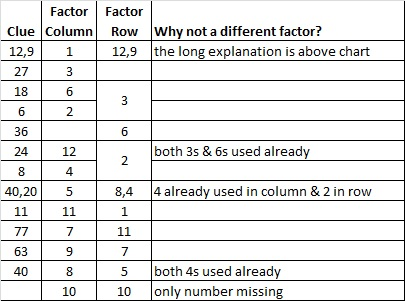# 1739 A Gift for a Valentine

### Today’s Puzzle:

Here’s a level 1 Valentine’s gift puzzle to start off the month of February. Place the numbers 1 to 10 in both the first column and the top row so that those numbers are the factors of the given number clues.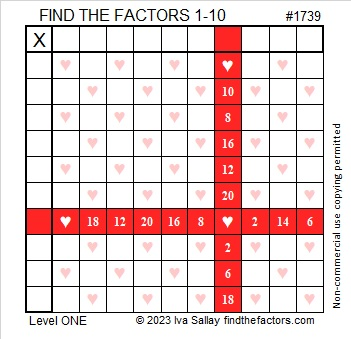And here’s a heartless copy of the same puzzle that may be more printer-friendly.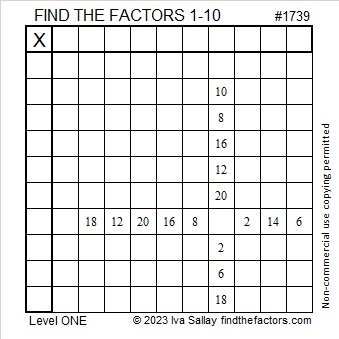### Factors of 1739:

Is 1739 a prime number? It isn’t divisible by 2 or 5.
1 + 7 + 3 + 9 = 20, so it isn’t divisible by 3.
1 – 7 + 3 – 9 = -12, so it isn’t divisible by 11.
√1739 is a little more than 41. Should I try dividing 1739 by every other prime number less than 41? I don’t know divisibility tricks for most of those prime numbers!

Here’s a shortcut I first wrote about in 341 is the smallest composite number that gives a false positive for this Quick Prime Number Test: First I type into my computer’s scientific calculator:
2  xʸ  1739  Mod  1739  =

If the answer on the calculator is anything other than “2”, then it can’t be a prime number. If it is “2”, it very likely is prime. As you can see by the screenshot of my calculator, 1739 is not prime!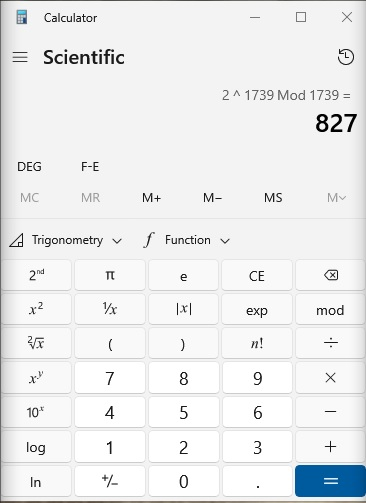• 1739 is a composite number.
• Prime factorization: 1739 = 37 × 47.
• 1739 has no exponents greater than 1 in its prime factorization, so √1739 cannot be simplified.
• The exponents in the prime factorization are 1 and 1. Adding one to each exponent and multiplying we get (1 + 1)(1 + 1) = 2 × 2 = 4. Therefore 1739 has exactly 4 factors.
• The factors of 1739 are outlined with their factor pair partners in the graphic below.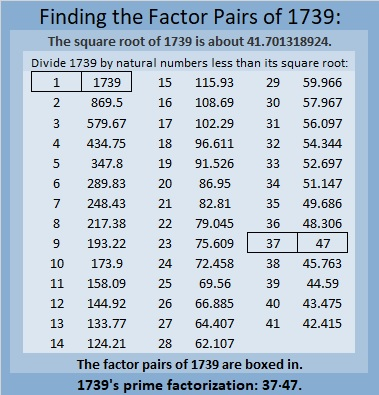### More About the number 1739:

1739 is the difference of two squares in two ways:
870² – 869² = 1739, and
42² – 5² = 1739.

That means 1739 is only 25 numbers away from the next perfect square!

# 1028 A Valentine Mystery

Valentine’s Day is almost here so I’ve made three Valentine related puzzles this week. I’ve labeled all of three of them Mystery Level because you might find some of them to be difficult. Use logic and an ordinary 12 × 12 multiplication table. I promise that each one of them can be solved, and I hope that you LOVE working on them! There will be some easier puzzles later on in the week.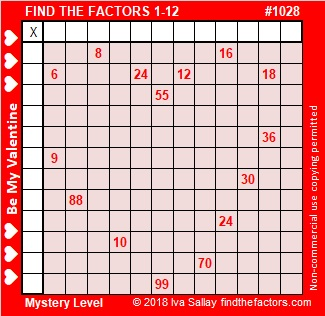Print the puzzles or type the solution in this excel file: 12 factors 1028-1034

Let me tell you a little about the number 1028:

32² + 2² = 1028 so 1028 is the hypotenuse of a Pythagorean triple:
128-1020-1028 which is 4 times (32-255-257) and can be calculated from 2(32)(2), 32² – 2², 32² + 2²

1028 is a palindrome when it is written in a couple of different bases:
404 in BASE 16 because 4(16²) + 4(1) = 4(257) = 1028
2G2 in BASE 19 (G is 16 base 10) because 2(19²) + 16(19) + 2(1) = 1028

• 1028 is a composite number.
• Prime factorization: 1028 = 2 × 2 × 257, which can be written 1028 = 2² × 257
• The exponents in the prime factorization are 2 and 1. Adding one to each and multiplying we get (2 + 1)(1 + 1) = 3 × 2  = 6. Therefore 1028 has exactly 6 factors.
• Factors of 1028: 1, 2, 4, 257, 514, 1028
• Factor pairs: 1028 = 1 × 1028, 2 × 514, or 4 × 257
• Taking the factor pair with the largest square number factor, we get √1028 = (√4)(√257) = 2√257 ≈ 32.062439# 796 and a Valentine

Here’s a puzzle you can print, cut out, and give as a valentine:You may know the divisibility rules for these powers of two:

• If the last digit of a number is divisible by 2, the whole number is divisible by 2.
• If the last two digits are divisible by 4, the whole number is divisible by 4.
• If the last three digits are divisible by 8, the whole number is divisible by 8.

But I’m going to apply some other time-saving but possibly more confusing divisibility rules to the number 796:

• 796 is divisible by 2 because 6 is an even number.
• 796 is divisible by 4 because even number 6 is NOT divisible by 4, and 9 is an odd number.
• 796 is NOT divisible by 8 because 96 is divisible by 8, and 7 is an odd number.

Because 796 is divisible by 4 but not by 8, it can be written as the sum of 8 consecutive numbers:

• 96 + 97 + 98 + 99 + 100 + 101 + 102 + 103 = 796

796 is also the sum of all the prime numbers from 113 to 149:

• 113 + 127 + 131 + 137 + 139 + 149 = 796

Here is the factoring information for 796:

• 796 is a composite number.
• Prime factorization: 796 = 2 x 2 x 199, which can be written 796 = (2^2) x 199
• The exponents in the prime factorization are 2 and 1. Adding one to each and multiplying we get (2 + 1)(1 + 1) = 3 x 2  = 6. Therefore 796 has exactly 6 factors.
• Factors of 796: 1, 2, 4, 199, 398, 796
• Factor pairs: 796 = 1 x 796, 2 x 398, or 4 x 199
• Taking the factor pair with the largest square number factor, we get √796 = (√4)(√199) = 2√199 ≈ 28.21347.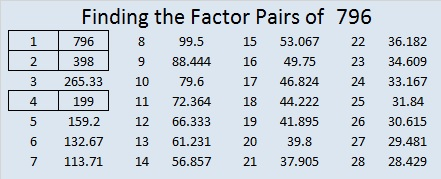# 62 and Love: Challenging But Worth It

62 is a composite number. 62 = 1 x 62 or 2 x 31. Factors of 62: 1, 2, 31, 62. Prime factorization: 62 = 2 x 31.62 is never a clue in the FIND THE FACTORS puzzles.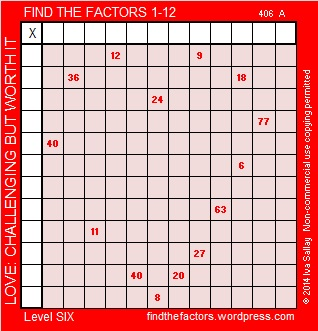All Previously Published PuzzlesHere is the logic to solve the puzzle & a chart showing one way to get the solution:

9 has to be the factor of both the 27 and the 63.  Therefore 3 x 6 = 18, (not 2 x 9).

3 is one of the factors of both the 18 and the 27.  Therefore 9 x 1 = 9, (not 3 x 3).